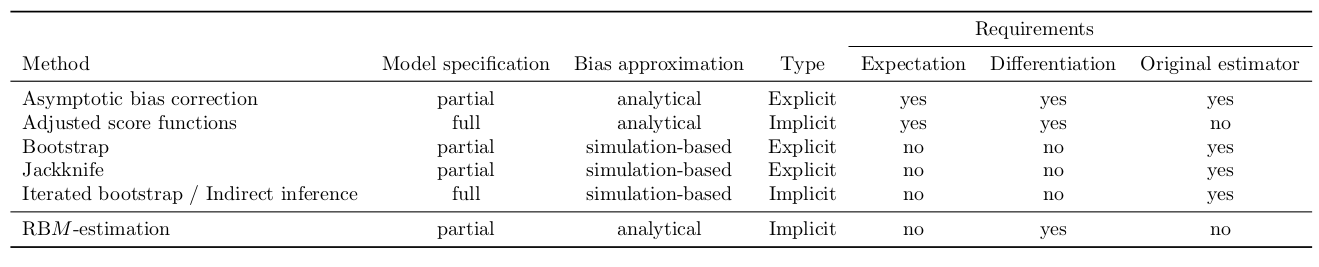# Bias reduction

## Estimating the bias of an estimator so as to subtract it off again

Trying to reduce bias in point estimators by, e.g. bootstrap. In, e.g. AIC we try to compensate for bias in the model selection. In bias reduction we try to eliminate it from our estimates.

This looks interesting: Kosmidis and Lunardon (2020)

The current work develops a novel method for the reduction of the asymptotic bias of M-estimators from general, unbiased estimating functions. We call the new estimation method reduced-bias M -estimation, or RBM -estimation in short. Like the adjusted scores approach in Firth (1993), the new method relies on additive adjustments to the unbiased estimating functions that are bounded in probability, and results in estimators with bias of lower asymptotic order than the original M -estimators. The key difference is that the empirical adjustments introduced here depend only on the first two derivatives of the contributions to the estimating functions, and they require neither the computation of cumbersome expectations nor the potentially expensive, calculation of M -estimates from simulated samples. Specifically, …, RBM -estimation

1. applies to models that are at least partially-specified;
2. uses an analytical approximation to the bias function that relies only on derivatives of the contributions to the estimating functions;
3. does not depend on the original estimator; and
4. does not require the computation of any expectations.Kosmidis’ comparison table

### No comments yet. Why not leave one?

GitHub-flavored Markdown & a sane subset of HTML is supported.